Question
1 views
What is the pH of 0.0050 M Ba(OH)2(aq)

2.00
11.70
2.30
12.00
check_circle

Step 1

pH scale is the measure of acidity or basicity for a solution. Acidity is defined by the presence of Hydrogen(H+) ion and basicity is defined by the presence of Hydroxide(OH-) ion in the particular solution.

For Acids it has a value lower than 7.

For Bases it has a value higher than 7.

For Neutral solution has a value 7.

Mathematically-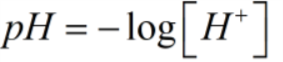Since,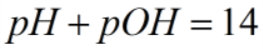Step 2

For bases calculate the Hydroxide(OH-) ion by using the relation-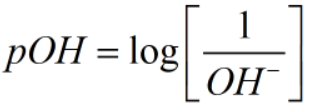Since,

Ba(OH)2(aq) completely dissociates can be represented as-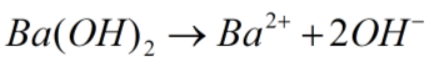Here, 2 moles of OH- ions are present therefore, it becomes

2×0.0050=0.01 moles of OH-

Step 3

To calculate pH first substitute value of OH- ion in pOH equation as mentioned above-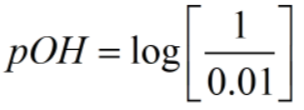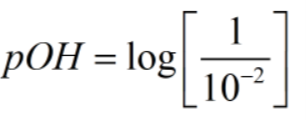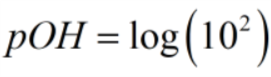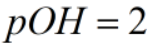...

### Want to see the full answer?

See Solution

#### Want to see this answer and more?

Solutions are written by subject experts who are available 24/7. Questions are typically answered within 1 hour.*

See Solution
*Response times may vary by subject and question.
Tagged in